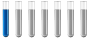## Help Finding Molarity Solution

Chemistry and homework help forum.

Organic Chemistry, Analytical Chemistry, Biochemistry, Physical Chemistry, Computational Chemistry, Theoretical Chemistry, High School Chemistry, Colledge Chemistry and University Chemistry Forum.

Share your chemistry ideas, discuss chemical problems, ask for help with scientific chemistry questions, inspire others by your chemistry vision!

Please feel free to start a scientific chemistry discussion here!

Discuss chemistry homework problems with experts!

Ask for help with chemical questions and help others with your chemistry knowledge!

Moderators: expert, ChenBeier, Xen

hp5182949
NewbiePosts: 3
Joined: Sat Oct 03, 2020 1:26 pm

### Help Finding Molarity Solution

In the reaction represented below, 1.456x10^5 ug of V2(CO3)5 can produce 25.00 mL of what molarity MgCO3 solution?
V = 50.94 g/mol
C = 12.01 g/mol
O = 16.00 g/mol

Equation: 3V2(CO3)5 + 5Mg3(PO4)2 ----------> 15MgCO3 + 2V3(PO4)5
ChenBeier
Distinguished MemberPosts: 432
Joined: Wed Sep 27, 2017 7:25 am
Location: Berlin, Germany

### Re: Help Finding Molarity Solution

1. Calculate the mass of Vanadiumcarbonate to mole
2. Calculate according the equation the mole of magnesiumcarbonate.
3. Calculate the molarity by using the given volume.
hp5182949
NewbiePosts: 3
Joined: Sat Oct 03, 2020 1:26 pm

### Re: Help Finding Molarity Solution

ChenBeier wrote: Mon Oct 05, 2020 10:37 pm 1. Calculate the mass of Vanadiumcarbonate to mole
2. Calculate according the equation the mole of magnesiumcarbonate.
3. Calculate the molarity by using the given volume.
Once I do that and then what?
ChenBeier
Distinguished MemberPosts: 432
Joined: Wed Sep 27, 2017 7:25 am
Location: Berlin, Germany

### Re: Help Finding Molarity Solution

Then you get the result.